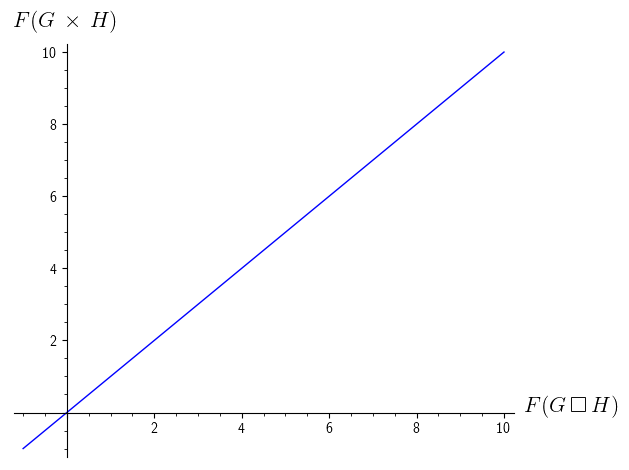# Problems with AMS symbols in Sage, Jupyter

How to display the square (\square) symbol in a graph (Jupyter, maybe not only). Why \boxtimes is too large?

plot(x, (x,-1,10), axes_labels=[r'$F(G\,\square\,H)$',r'$F(G\,\times\,H)$']).show()

plot(x, (x,-1,10), axes_labels=[r'$F(G\,\square\,H)$',r'$F(G\,\times\,H)$']).show()


I tried \box \square \emptybox etc. and

from sage.misc.latex import latex_extra_preamble


I get:

ValueError: F(G\,\square\,H) ^ Unknown symbol: \square (at char 5), (line:1, col:6)

edit retag close merge delete

Sort by » oldest newest most voted

Hello, @SYLA. As SageMath uses Matplotlib as its backend for plotting, you should import the amssymb package using Matplotlib itself. The only way I can find to do this is the following:

from matplotlib import rc
rc('text', usetex=True)
rc('text.latex', preamble=r'\usepackage{amssymb}')
plot(x, (x,-1,10), axes_labels=[r'$F(G\,\square\,H)$',r'$F(G\,\times\,H)$']).show()


Here is the result:First, we import rc from Matplotlib, which is used to specify configurations. In the second line, we use rc to specify the use of LaTeX as default renderer for text. The third line indicates that we want to add the amssymb package, which defines the \square command you need. Finally, we use Sage's command for plotting.

The interesting thing is that Sage first processes the plot command, and then passes it to Matplotlib for plotting, and that is when our new configurations take effect.

I hope this helps!

more

That is a very interesting answer. Should this maybe be reported as a bug in Sage so that people can pass more options to plot?

Hello, @kcrisman. I agree, it should be reported as a bug. However, I would like to wait a little in order to see if an alternative is proposed. Maybe there is some command for this purpose which I am not aware of. I would like to ask @john-palmieri or any of the experts in Sage about this, before posting a bug report.